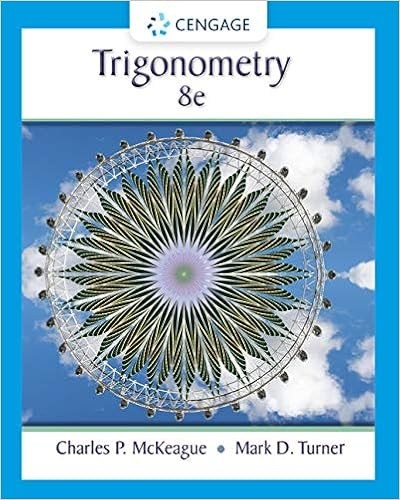# MAT 126 FE Practice Exam.pdf - Stony Brook STATE UNIVERSITY...

• Test Prep
• 9

This preview shows page 1 - 2 out of 9 pages.

##### We have textbook solutions for you!
The document you are viewing contains questions related to this textbook.The document you are viewing contains questions related to this textbook.
Chapter A / Exercise 21
Trigonometry
McKeague/TurnerExpert Verified
MAT 126 Final Exam Review Page 1of 9Stony Brook STATE UNIVERSITY OF NEW YORK MAT 126 Practice Final Exam Problem 1 2 3 4 5 6 7 8 9 10 11 13 14 15 Total Percent Score Last Name: _____________________ First Name:_________________ Recitation #: _______ (See below) Directions:Answer all questions in the space provided. You may use the blank backs of pages for scrap. No other paper is permitted. Show ALL relevant work. Calculators are not to be used. Circle your final answers. Show all work in the space provided. Be sure that you don’t have answers to any question in more than one place. Answers without the required work will receive no credit. Simplify your answers. Each numbered question is worth 10 points. Note that the total number of points is 150. 1. The graph of ࠵?(࠵?)is given at the right. Each square is 1 unit by 1 unit. If ࠵?(࠵?) = ∫ ࠵?(࠵?)࠵?࠵?࠵?0find the following: a) ࠵?(4)b) ࠵?(7)c) For what value of x does g have a maximum value? ࠵?࠵?࠵?2࠵? =12(1 + ࠵?࠵?࠵?2࠵?) ࠵?࠵?࠵?2࠵? =12(1 − ࠵?࠵?࠵?2࠵?)
##### We have textbook solutions for you!
The document you are viewing contains questions related to this textbook.The document you are viewing contains questions related to this textbook.
Chapter A / Exercise 21
Trigonometry
McKeague/TurnerExpert Verified
•••